## Transient Analysis of Induction Motor:

Usefulness of analysis of transient operating conditions of a drive, e.g. starting, braking, load changing, speed changing, etc. is already explained. A rigorous analysis of transient operation of an induction motor drive, can be done only by the d-q axis model involving long calculations. A simple method of analysis, with satisfactory accuracy for most applications is obtained by using steady-state torque relations. Such an analysis is based on the assumption that electrical time constants can be neglected, as they are very small compared to mechanical time constant. Thus, we can write following equation for Transient Analysis of Induction Motor drive: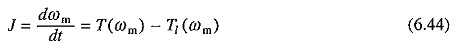Equation (6.44) can.be evaluated graphically to obtain ωm vs t curve, and energy losses in motor and external rotor resistance using the method already explained. This approach is general and can be employed for any Transient Analysis of Induction Motor as long as steady-state speed-torque curves of motor [T(ωm)] and load [T1m)] are known. Approximate analytical methods are presented below.

### Starting and Plugging:

For starting and plugging operation of machine, torque is given by Eq. (6.15). Substituting from Eq. (6.15) into (6.44) yields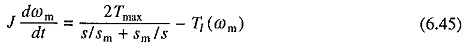In some cases, Eq. (6.45) will be in integrable form, and therefore, can be solved analytically. It is useful to examine the transients for starting and plugging operations when operating on no load. Thus, from Eq. (6.45) for no load operation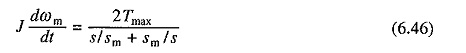Differentiating Eq. (6.3) gives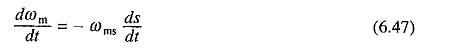Substituting from Eq. (6.47) into (6.46) and rearranging the terms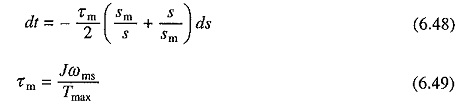τm is the mechanical time constant of motor. It is defined as the time taken by motor to reach its synchronous speed from standstill under constant accelerating torque equal to the maximum torque of the motor.

From Eq. (6.48), time required to start an induction motor on no load is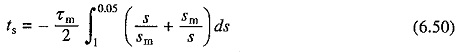When operating on no load, steady-state is reached when s = 0. Thus during starting slip changes from 1 to 0. However, if (6.50) is integrated for s = 1 to s = 0 an infinite value is obtained for starting time. As explained, when final speed is the steady-state equilibrium speed, transients are considered to be over when 95% range of speed is covered. Therefore, in Eq. (6.50) integration is done from s = 1 to s = 0.05. Solving (6.50) gives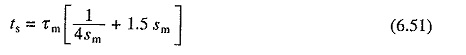Thus starting time is a function of sm. Starting time has a minimum value of 1.22τm at sm = 0.4. From Eq. (6.12), when Rs is negligible, rotor resistance required to start the motor in minimum time is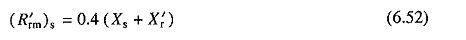From Eq. (6.48), time required for stopping by plugging, when initially running at synchronous speed, can be expressed as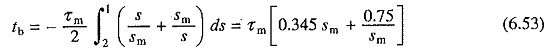Stopping time is again a function of sm. It has a minimum value of 1.027τm at sm = 1.47. Corresponding value of rotor resistance is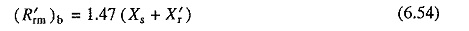Similarly, from Eq. (6.48), time required for speed reversal by plugging when running on no load is given by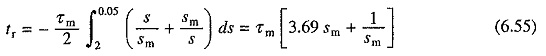Minimum time for reversal is thus 2.88τm and corresponding value of sm is 0.52. Rotor resistance required for speed reversal by plugging in minimum time is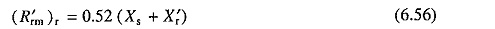### Calculation of Energy Losses:

Let us next obtain expressions for energy loss in motor windings for starting and plugging operations. The rotor winding loss for starting can be written as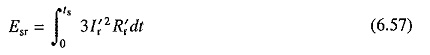Substituting from Eqs. (6.5) and (6.11) gives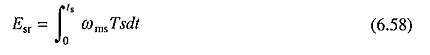As the machine is operating under no load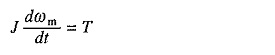Substituting from Eq. (6.47)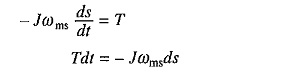or

Substituting in (6.58) gives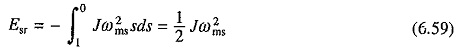It is interesting to note that rotor winding energy loss is equal to the kinetic energy stored in moving parts at completion of the starting process, and it is independent of the starting time or rotor resistance. However, if an external resistance is connected in rotor circuit only a part of this loss is used to heat the motor. Energy loss in stator winding, neglecting magnetizing current is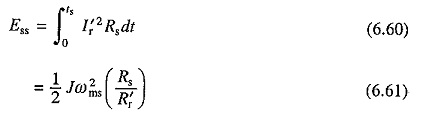Hence, total winding loss during starting under no load is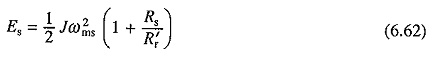Proceeding s milady, rotor winding loss during stopping by plugging under no load can be written as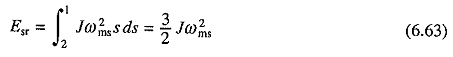Equation (6.59) suggests that rotor winding loss can be reduced when started by using methods based on the variation of synchronous speed. As an example let us consider a motor with an arrangement for doubling the pole number. Let it be started with higher pole number for which the synchronous speed is ωms/2. Then, from (6.59) rotor copper loss for change of speed from 0 to ωms/2 will be Jω2ms/8. Now the pole number is lowered. Consequently, rotor copper loss for speed range ωms/2 to ωms will be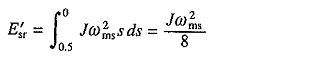Thus, total rotor winding loss is Jω2ms/4, which is one-half of the copper loss when there is no provision for doubling the pole number.

Scroll to Top